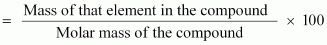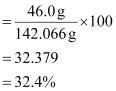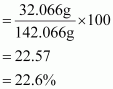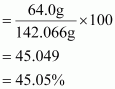# Calculate the mass per cent of different elements present in sodium sulphate(Na2SO4).

10 years ago

The molecular formula of sodium sulphate is.

Molar mass of  = [(2 × 23.0) + (32.066) + 4 (16.00)]

= 142.066 g

Mass percent of an element∴ Mass percent of sodium:Mass percent of sulphur:Mass percent of oxygen: